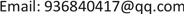﻿ 电价改革条件下电网投资策略研究 Research on the Investment Strategy of Power Grid under the Condition of Electricity Price Reform

Journal of Electrical Engineering
Vol.06 No.01(2018), Article ID:24270,7 pages
10.12677/JEE.2018.61014

Research on the Investment Strategy of Power Grid under the Condition of Electricity Price Reform

Guangliang Yu, Nan Zheng, Juannan Liu, Jinfeng Wang, Chunyang Wu, Huimin Ren

State Grid Shaanxi Electric Power Company Economic Research Institute, Xi’an ShaanxiReceived: Mar. 8th, 2018; accepted: Mar. 22nd, 2018; published: Mar. 29th, 2018ABSTRACT

Many provinces have carried out the pilot project on the reform of the power transmission and distribution price with the deepening of the reform of the power price in China in recent years. After the electricity price reform, the government approves the power transmission and distribution price, strengthens the supervision and cost constraint for the power grid enterprises, raises the demand for the power grid’s economy, and changes the profit mode of the power grid enterprises. In order to cope with the impact of the reform of power transmission and distribution price, according to calculation method and pricing mechanism in the Provincial Power Grid Transmission and Distribution Pricing Measure (Trial), build power transmission and distribution pricing model. From three aspects of investment scale, investment structure, investment timing, analyses the impact of the reform of power transmission and distribution price for power grid investment, and puts forward some corresponding strategies.

Keywords:Reform of Electricity Price, Power Grid Investment, Investment Scale, Investment Structure, Investment Timing1. 引言

2. 电价改革对电网投资规模的影响及应对策略

2.1. 投资规模模型的建立

${P}_{t}=\frac{{I}_{t}}{{Q}_{t}}$ (1)

$|\frac{\left({Q}_{s}-{Q}_{t}\right){P}_{t}}{{P}_{t}{Q}_{t}}|=|\frac{{Q}_{s}}{{Q}_{t}}-1|\ge 6%$ (2)

2.2. 对策建议

3. 电价改革对电网投资结构的影响及应对策略

3.1. 不同电压等级输配电成本模型

${\eta }_{NPV}\left({I}_{L}^{u}\right)={\sum }_{t=1}^{n}\frac{{I}_{t,L}^{u}}{{\left(1+i\right)}^{n}}$ (3)

${\eta }_{NPV}\left({I}_{T}^{u}\right)={\sum }_{t=1}^{n}\frac{{I}_{t,T}^{u}}{{\left(1+i\right)}^{n}}$ (4)

${S}_{AICL}^{u}={\eta }_{NPV}\left({I}_{L}^{u}\right)/{\eta }_{NPV}\left(\Delta {S}^{u}\right)$ (5)

${S}_{AICT}^{U}={\eta }_{NPV}\left({I}_{T}^{u}\right)/{\eta }_{NPV}\left(\Delta {P}_{DT}^{u}\right)$ (6)

${A}_{L}^{u}={S}_{AICL}^{u}\left(A/P,i,n\right)+M={S}_{AICL}^{u}\frac{i{\left(1+i\right)}^{n}}{{\left(1+i\right)}^{n}-1}+{S}_{AICL}^{u}\theta$ (7)

${A}_{T}^{u}={S}_{AICT}^{U}\left(A/P,i,n\right)+M={S}_{AICT}^{U}\frac{i{\left(1+i\right)}^{n}}{{\left(1+i\right)}^{n}-1}+{S}_{AICT}^{u}\theta$ (8)

3.2. 对策建议Table 1. Formula (3)、(4)、(5)、(6)、(7)、(8) parameter table

4. 电价改革对电网投资时序的影响及应对策略

4.1. 对投资时序的影响

4.1.1. 基于输配电量变动对投资时序的影响

4.1.2. 基于折旧的变动对投资时序的影响

4.1.3. 基于通货膨胀率的变动对投资时序的影响

4.2. 对策建议

5. 总结

Research on the Investment Strategy of Power Grid under the Condition of Electricity Price Reform[J]. 电气工程, 2018, 06(01): 127-133. https://doi.org/10.12677/JEE.2018.61014

1. 1. 徐帅, 孙媛媛, 叶泽, 等. 输配电价改革背景下省级电网企业财务管理的转型[J]. 中国电力企业管理, 2017(7): 40-45.

2. 2. 李江涛. 对《省级电网输配电价定价办法(试行)》的认识与思考[J]. 中国电力企业管理, 2017(7): 46-49.

3. 3. 涂俊华. 新电改形势下对电网建设投资的几点思考[J]. 机电信息, 2017(24): 154-155.

4. 4. 季立伟, 杨丽萍, 费改英. 电网工程投资影响因素分析及预测[J]. 中国电力企业管理, 2016(6): 85-92.

5. 5. 赵会茹, 杨璐, 李春杰, 等. 基于协整理论和误差修正模型的电网投资需求预测研究[J]. 电网技术, 2011(9): 193-198.

6. 6. 陈煜, 刘敦南, 王宝, 等. 输配电价改革环境下电网最大投资能力研究[J]. 水电能源科学, 2017(12): 212-216.

7. 7. 杜宇. 价格监管的重大创新[J]. 中国招标, 2017(5): 12-13.

8. 8. 国网湖北省电力公司经济技术研究院电价改革课题组. 深圳输配电价改革试点启示[J]. 中国电力企业管理, 2015(13): 58-63.

9. 9. 中华人民共和国国家发展和改革委员会. 国家发展改革委关于印发《省级电网输配电价定价办法(试行)》的通知[EB/OL]. http://www.ndrc.gov.cn/zcfb/zcfbtz/201701/t20170104_834311.html, 2016-12-22.

10. 10. 沈运帷, 李扬, 焦系泽, 等. 新电改背景下需求响应成本效益分析及其融资渠道[J]. 电力自动化设备, 2017(9): 124-130.

11. 11. 刘思强, 叶泽, 范先国, 等. 定价参数对输配电价的影响及调控模型[J]. 电力系统自动化, 2017, 41(24): 58-65.

12. 12. 陈锋, 张宇田. 输配电价改革对电网企业财务管理的影响及对策分析[J]. 商业经济, 2017(1): 55-56.

13. 13. 韩勇, 田闻旭, 谭忠富. 基于长期边际成本的不同电压等级输配电价定价模型及其应用[J]. 电网技术, 2011, 35(7): 175-180.

14. 14. 董红, 刘文革, 朱志芳. 输配电价改革对广州电网规划的影响及应对策略[J]. 广东电力, 2016, 29(5): 42-45, 66.

15. 15. 钱唯克, 钟彬, 陈启昉, 等. 电力体制改革对电网企业投资管理的影响及对策[J]. 经济与管理, 2017(10): 105-108.